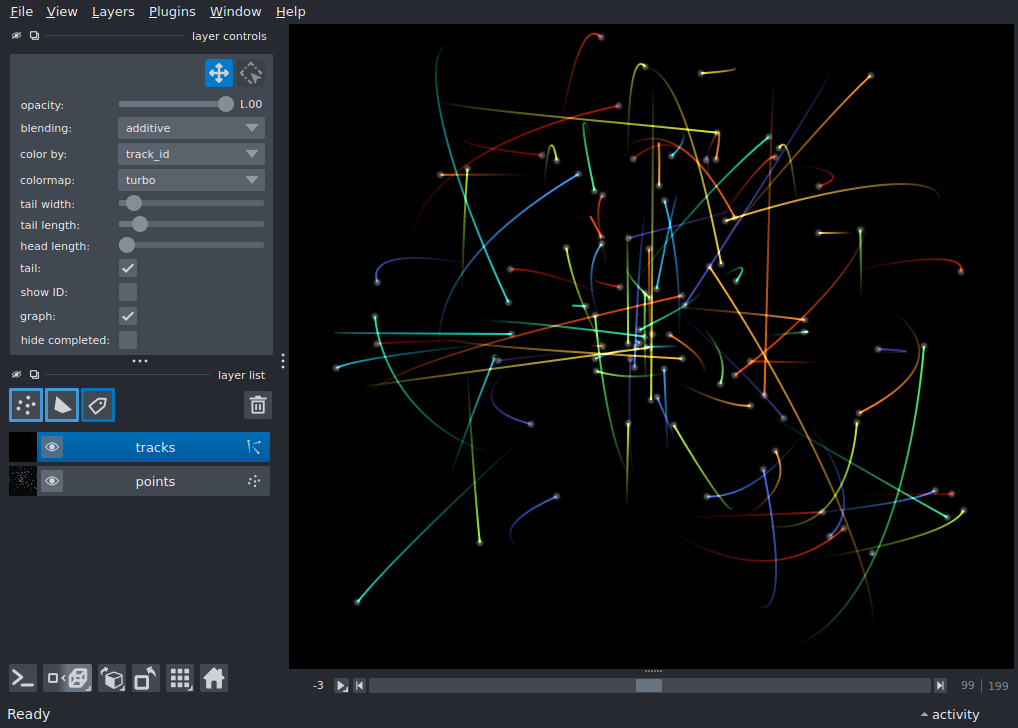# Tracks 3D¶```import numpy as np

import napari

def lissajous(t):
a = np.random.random(size=(3,)) * 80.0 - 40.0
b = np.random.random(size=(3,)) * 0.05
c = np.random.random(size=(3,)) * 0.1
return (a[i] * np.cos(b[i] * t + c[i]) for i in range(3))

def tracks_3d(num_tracks=10):
""" create 3d+t track data """
tracks = []

for track_id in range(num_tracks):

# space to store the track data and features
track = np.zeros((200, 10), dtype=np.float32)

# time
timestamps = np.arange(track.shape)
x, y, z = lissajous(timestamps)

track[:, 0] = track_id
track[:, 1] = timestamps
track[:, 2] = 50.0 + z
track[:, 3] = 50.0 + y
track[:, 4] = 50.0 + x

# calculate the speed as a feature

speed = np.sqrt(gx ** 2 + gy ** 2 + gz ** 2)
distance = np.sqrt(x ** 2 + y ** 2 + z ** 2)

track[:, 5] = gz
track[:, 6] = gy
track[:, 7] = gx
track[:, 8] = speed
track[:, 9] = distance

tracks.append(track)

tracks = np.concatenate(tracks, axis=0)
data = tracks[:, :5]  # just the coordinate data

features = {
'time': tracks[:, 1],
'speed': tracks[:, 8],
'distance': tracks[:, 9],
}

graph = {}
return data, features, graph

tracks, features, graph = tracks_3d(num_tracks=100)
vertices = tracks[:, 1:]

viewer = napari.Viewer(ndisplay=3)